Page 2This text is meant to accompany class discussions. It is not everything there is to know about uniform circular motion. It is meant as a  prep for class. More detailed notes and examples are given in the class notes, presentations, and demonstrations (click here.)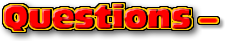Click for the questions that go with this reading
The formulas

Physicists are all about making predictions by creating a model for understanding what happens. One of the models is the conceptual model that shows how the different vectors connect to find the final answer. (See the example on the previous page.) A second model involves uses displacement, velocity and/or acceleration versus time graphs. A final model is to create a series of functions to determine relationships between the variables used in describing the motion of the bodies.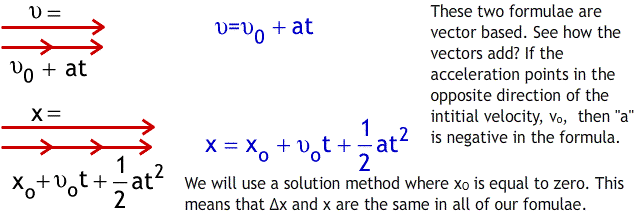The average velocity is defined as,These are two formulae that you have probably seen before, x/t is from the distance = (rate)(time) formula from algebra I. The other average equation is the definition of finding the average of any two numbers: Add up the two numbers and divide by two. However, the power of the of equation is not in calculating the average velocity but in relating the two ways the average velocity is calculated.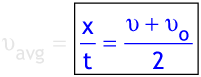The final functions describes the final velocity as a function of displacement. This is found by eliminating time.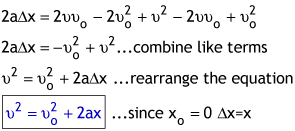These four equations will be used to solve problems that describe the motion of a moving body. Remember the assumption is that the acceleration is constant.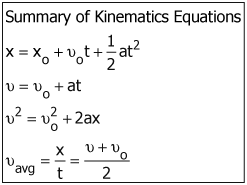by Tony Wayne ...(If you are a teacher, please feel free to use these resources in your teaching.)

 The owner of this website does not collect cookies when the site is visited. However, this site uses and or embeds Adobe, Apple, GoDaddy, Google, and YouTube products. These companies collect cookies when their producs are used on my pages. Click here to go to them to find out more about how they use their cookies. If you do not agree with any of their policies then leave this site now.